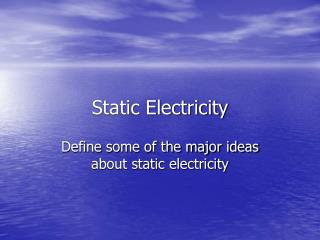DownloadDownload PresentationStatic Electricity

# Static Electricity

Download Presentation## Static Electricity

- - - - - - - - - - - - - - - - - - - - - - - - - - - E N D - - - - - - - - - - - - - - - - - - - - - - - - - - -
##### Presentation Transcript

1. Static Electricity Define some of the major ideas about static electricity

2. Static Electricity • Electricity “exists” because of two types of charge • Charge is (+) or (-) • Fundamental unit is the charge of one electron = one proton = 1.6 x 10-19C • Unit of charge is the Coulomb. One coulomb is a boatload of electrons • Like charges repel, opposites attract • Charge is Quantized, you can’t have ½ of an electron charge. Can’t break the charge on one electron any smaller.

3. Static Electricity • Ways to charge objects include: • Friction, like rubbing the fur on plastic • Conduction, touching a charged object to another object • Induction, like in the lab (which maybe didn’t work too well). Bring a charged object next to a conductor and then “ground” the conductor, leaving it charged.

4. Static Electricity • Insulators: electrons are held tightly and can’t move around easily, so electricity doesn’t move through them. Examples are rubber, plastic, glass • Conductors: charge (electrons) moves easily. Examples are metals: copper, aluminum, gold

5. Static Electricity • Electric Force • You saw the tape attract or repel, what causes things to move? (rhymes with horse) • There is an electric force between any 2 charged objects called the Coulomb Force • Force depends on the amount of charge on each object and on the distance between them. This force is analogous to the Universal Gravitational Force. Can you write the equation for these?

6. Electric Force • Coulomb’s law for electric force • F = (kq1q2)/r2 • q = amount of charge in coulombs • r = distance between charged objects • k = Coulomb constant = 9x109 • The force is the same on both charged objects (remember forces exist in pairs, Newton’s 3rd law)

7. Electric Force • Like charges repel, opposites attract

8. Electric Force • The electric force is conceptually much bigger than the gravitational force. • Why don’t we see the “electric force” much? • Objects in our world are typically neutral (even number of electrons and protons)

9. Electric Field • Much like the concept of an invisible Gravitational Field, there exists an invisible electric field around any charged object. • The Electric field is defined as the Force that another charged object (test charge) would feel in the presence of the source charged object divided by the amount of the charge on the test charge • E = F/q = kQq/r2q = kQ/r2

10. Electric Field Lines • Electric field lines surround a charged object and point in the direction that a positive (+) test charge would be forced to move

11. Electric field lines